Question

5) A solenoid comprises 500,000 turns of wire and is 10 cm long and 1.0 cm in radius.
a) Find the resultant magnetic field inside the coil if a current of 1 μA flows through the wire.
b) How would this change if the core is filled with mumetal (μ = 300 μ0 ).

6) The solenoid of Q5 is placed in a region where the perpendicular magnetic field strength is varying sinusoidally by 20 μT at a rate of 50 Hz.
Find the resultant emf induced in the coil.​​​​​​​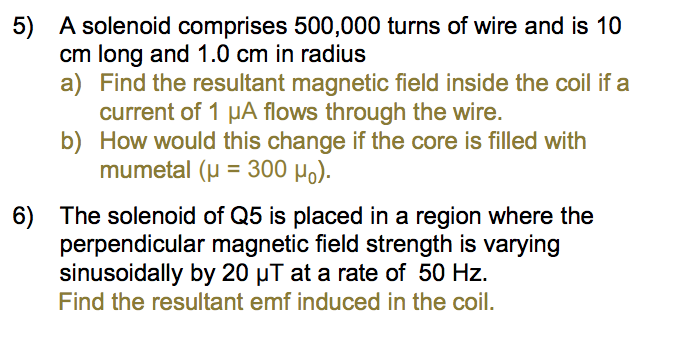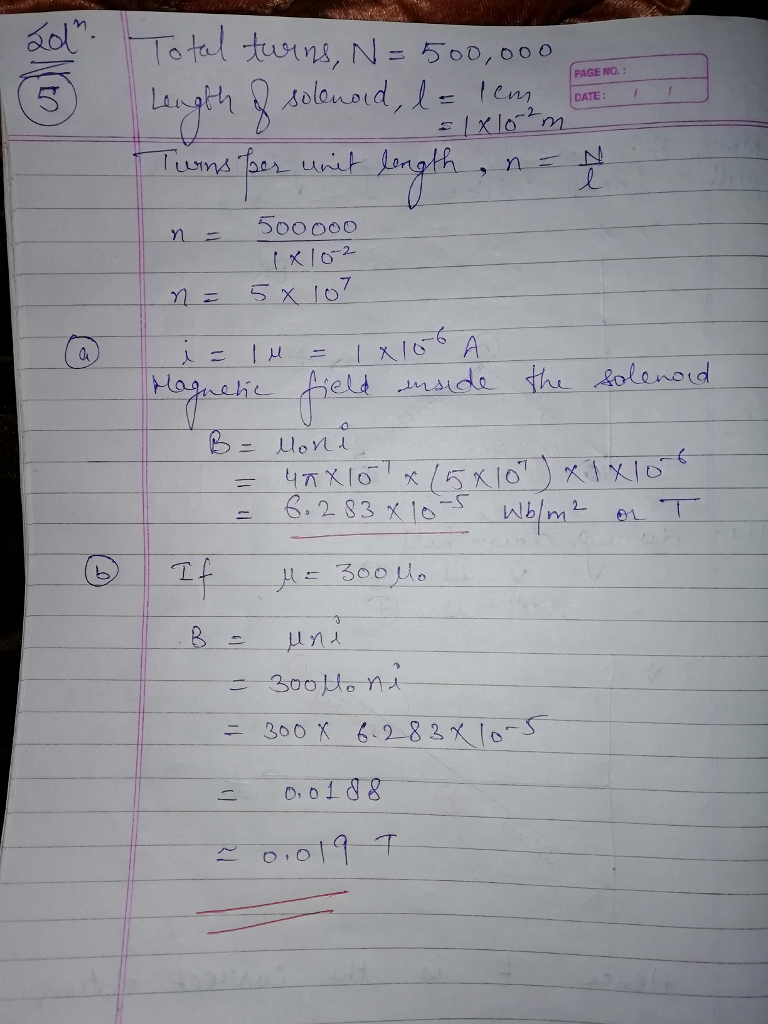#### Earn Coins

Coins can be redeemed for fabulous gifts.

Similar Homework Help Questions
• ### A 5.0 cm long solcnoig has Iboo turns of wire and carries a current of 70...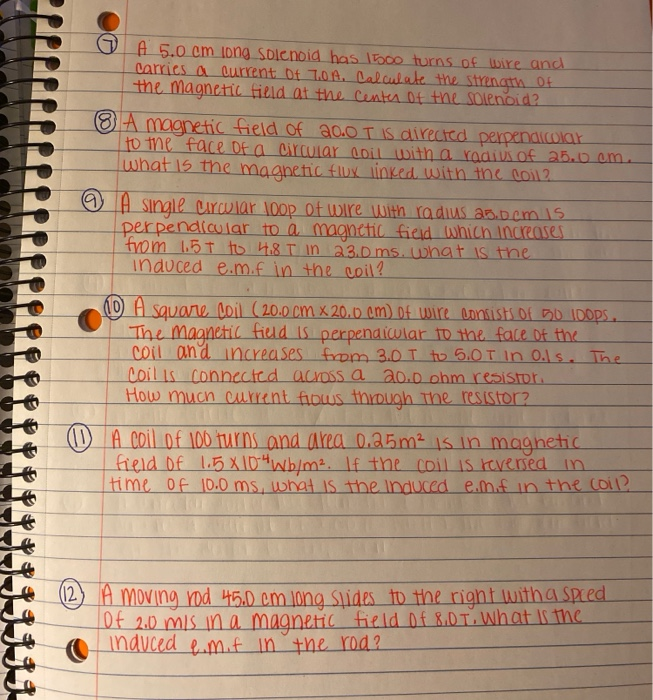A 5.0 cm long solcnoig has Iboo turns of wire and carries a current of 70 4. Calculate the strength of the magnetic field at the center of the solenoid? A magnetic field of 20.0 T is directed perpendicolar to the face of a circular coil with a radius of 25.0 om. what is the magnetic flux linked with the coil2 © A single urcular 100p of wire with radius ab.bcm 15 perpendicular to a magnetic field which increases from...

• ### An iron-core solenoid is 33 cm long and 1.9 cmin diameter, and has 690 turns of...

An iron-core solenoid is 33 cm long and 1.9 cmin diameter, and has 690 turns of wire. The magnetic field inside the solenoid is 1.9 T when 51 A flows in the wire.What is the permeability μ at this high field strength? μ=_____T⋅m/A?

• ### 3. Find the magnitude of the induced electric field outside a long solenoid at a distance rZR from its central axis if the solenoid is of radius R and hasn turns of wire per unit length and carri...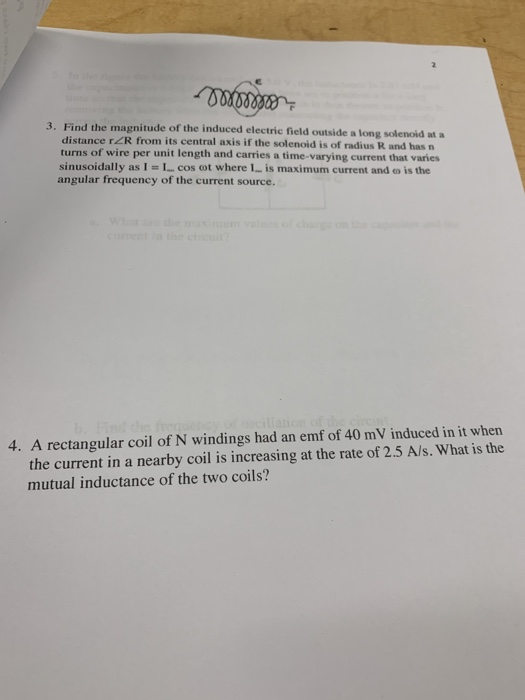3. Find the magnitude of the induced electric field outside a long solenoid at a distance rZR from its central axis if the solenoid is of radius R and hasn turns of wire per unit length and carries a time-varying current that varies sinusoidally as l = 1-cos cot where I-is maximum current and ω is the angular frequency of the current source. 4. A rectangular coil of N windings had an emf of 40 mV induced in it wher...

• ### A long solenoid of radius R 10 cm has n 100 turns/cm and carries a time-varying...A long solenoid of radius R 10 cm has n 100 turns/cm and carries a time-varying current that varies sinusoidally as IImax cos (2d), where Imax = 10 A is the maximum current andf-60 Hz is the frequency of the alternating current source. What is the maximum magnitude of the induced electric field inside the solenoid, a distance , 1.3 cm from its axis? Path of integration

• ### An iron-core solenoid is 43 cm long and 21 cm in diameter and has 570 turns...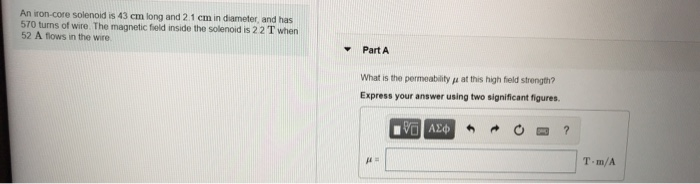An iron-core solenoid is 43 cm long and 21 cm in diameter and has 570 turns of wire. The magnetic field inside the solenoid is 22 T when 52 A flows in the wire Part A What is the permeability pe at this high field strength? Express your answer using two significant figures. VD AED O ? T.m/A

• ### #16 A 22.0-cm-diameter coil consists of 28 turns of circular copper wire 2.6 mm in diameter....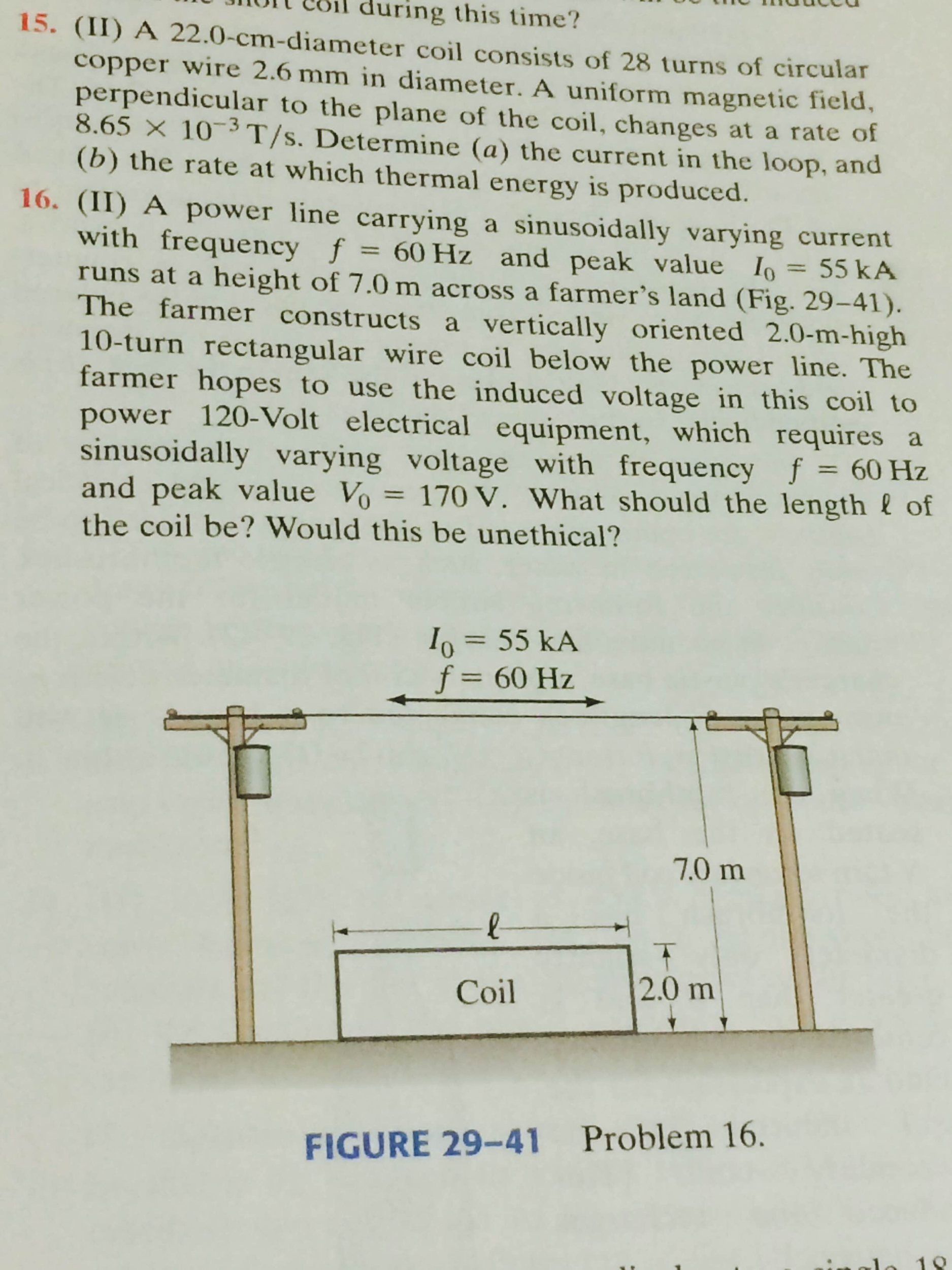#16 A 22.0-cm-diameter coil consists of 28 turns of circular copper wire 2.6 mm in diameter. A uniform magnetic field, perpendicular to the plane of the coil, changes at a rate of 8.65 Times 10^-3 T/s. Determine the current in the loop, and the rate at which thermal energy is produced. A power line carrying a sinusoidally varying current with frequency f = 60 Hz and peak value I_0 = 55 kA runs at a height of 7.0 m across...

• ### Problem 3 Part A A copper wire with resistance 0.010 Ω is shaped into a complete circle of radius R 10 cm and placed in a long solenoid so that the axis of the solenoid and the axis of the wire loop...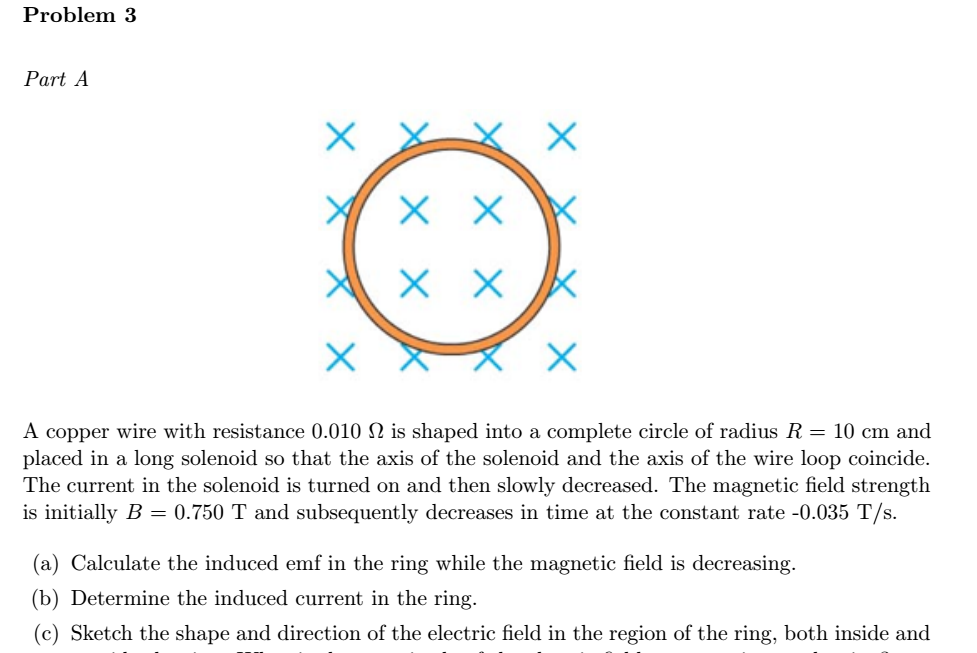Problem 3 Part A A copper wire with resistance 0.010 Ω is shaped into a complete circle of radius R 10 cm and placed in a long solenoid so that the axis of the solenoid and the axis of the wire loop coincide. The current in the solenoid is turned on and then slowly decreased. The magnetic field strength is initially B 0.750 T and subsequently decreases in time at the constant rate -0.035 T/s. (a) Calculate the induced emf...

• ### 4) Induction Effect. A very long solenoid of radius R-2.5 cm has n 5 turns/cm. Starting...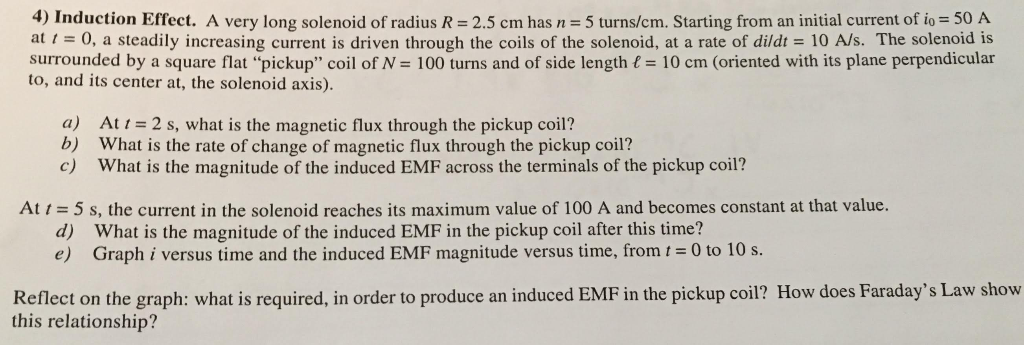4) Induction Effect. A very long solenoid of radius R-2.5 cm has n 5 turns/cm. Starting from an initial current of io 50 A at 1 = 0, a steadily increasing current is driven through the coils of the solenoid, at a rate of didt = 10 A/s. The solenoid is surrounded by a square flat "pickup" coil of N= 100 turns and of side lengthに10 cm (oriented with its plane perpendicular to, and its center at, the solenoid axis)....

• ### Problem 5. (4 points) Electric field induced by a changing magnetic field in a solenoid. long...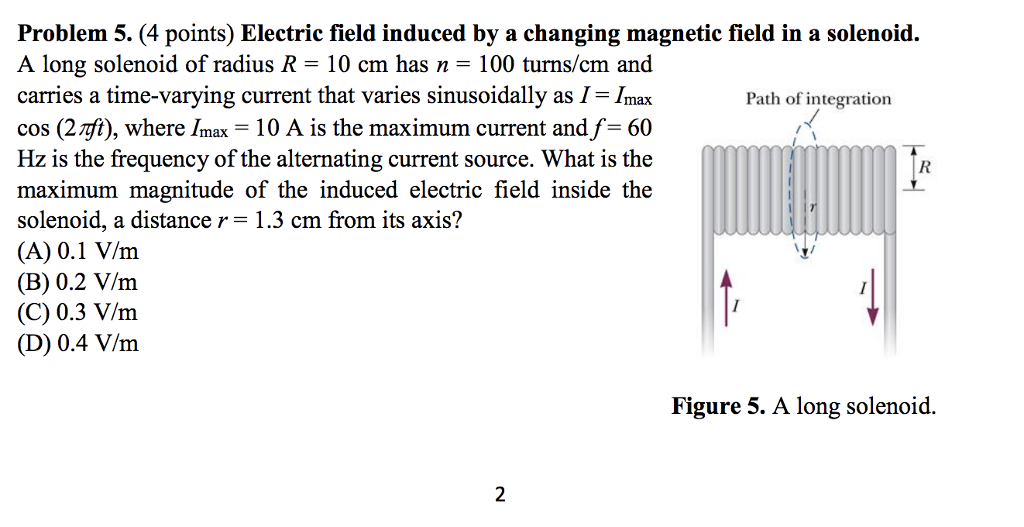Problem 5. (4 points) Electric field induced by a changing magnetic field in a solenoid. long solenoid of radius R = 10 cm has n = 100 turns/cm and carries a time-varying current that varies sinusoidally as I- Imax cos (2rft), where Imax- 10 A is the maximum current and f-60 Hz is the frequency of the alternating current source. What is the maximum magnitude of the induced electric field inside the solenoid, a distance r- 1.3 cm from its...

• ### A solenoid of length 3.00 cm and radius 0.950 cm has 37 turns. If the wire...

A solenoid of length 3.00 cm and radius 0.950 cm has 37 turns. If the wire of the solenoid has 1.35 amps of current, what is the magnitude of the magnetic field inside the solenoid? magnitude of the magnetic field: 2.09 ×10 −3 T Ignoring the weak magnetic field outside the solenoid, find the magnetic energy density inside the solenoid. magnetic energy density: 1.738 J/m3 What is the total magnetic energy inside the solenoid? total magnetic energy: ?? Need help...